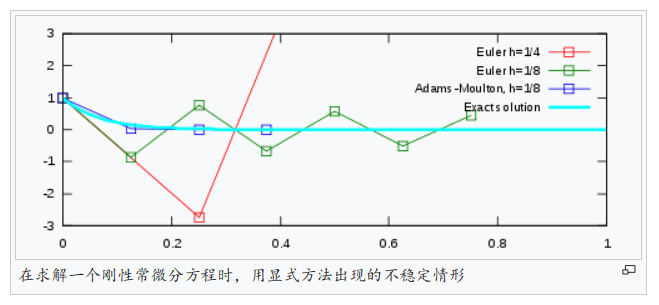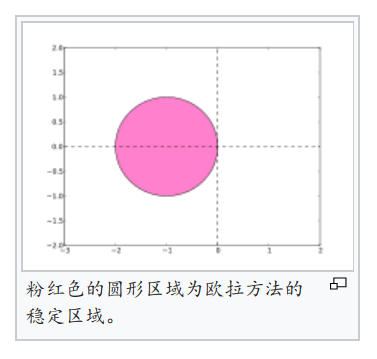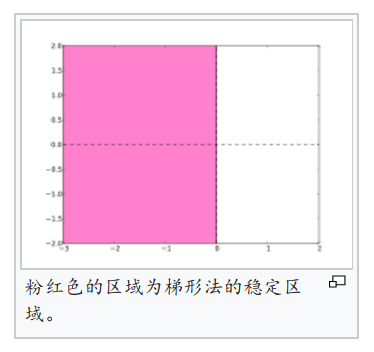# 刚性方程

### 2. 举例

$\begin{array}{c} y^{'} = -15y(t), t \geq 0, y(0) = 1 \end{array}$

$\begin{array}{c} y(t) = e^{-15t} \end{array}$• 若以欧拉方法来求数值解，则使用不同的步长将会得到不同的结果。第一种，步长${h=1/4}$的欧拉法强烈的震荡并且很快离开了图的边界。当将步长减半为 ${h=1/8}$时，得到的结果在图的范围以内。但是它依然在${0}$附近震荡，并且不可能表示精确的解。

• 而梯形法（即两阶段亚丹士-莫耳吞法）表达为

$\begin{array}{c} y_{n+1} = y_n + {1 \over 2}h(f(t_n, y_n) + f(t_{n+1}, y_{n+1})) \end{array}$

### 4. 稳定区域

• 将龙格－库塔法应用至测试方程 ${y^{'} = ky}$ ，可以得到如 ${y_{n+1} = \phi(hk)y_n}$ 的形式，并可归纳出 ${y_n = (\phi(hk))^ny_0}$ ，其中 $\phi$ 称为稳定性函数。因此

$\begin{array}{c} \lim_{n \rightarrow \infty}y_n = 0 \end{array}$

$\begin{array}{c} |\phi(hk)| \lt 1 \end{array}$

• 若一个方法的稳定区域包含 ${ \{z \in \mathbb {C} |\,\,\mathrm {Re} (z) \lt 0 \} }$（即左半平面），则称该方法为A-稳定。